Drug classification and concentration estimation from Raman spectra

Current events on this problem
Keywords
drug_spectra_starting_kit

Paris Saclay Center for Data Science

RAMP on qualitative and quantitative non-invasive monitoring of anti-cancer drugs

Camille Marini (LTCI/CNRS), Alex Gramfort (LTCI/Télécom ParisTech), Sana Tfaili (Lip(Sys)²/UPSud), Laetitia Le (Lip(Sys)²/UPSud), Mehdi Cherti (LAL/CNRS), Balázs Kégl (LAL/CNRS)

Introduction

Chemotherapy is one of the most used treatment against cancer. It uses chemical substances (chemotherapeutic agents) which kill cells that divide too quickly. These chemical substances are often diluted in a particular solution and packaged in bags, diffusers, or syringes, before being administered. Wrong medication (wrong chemotherapeutic agent or wrong concentration) can have major impacts for patients. To prevent wrong medication, some recent French regulations impose the verification of anti-cancer drugs before their administration. The goal is to check that they contain the good chemotherapeutic agent with the good dosage.

Raman spectroscopy could be used to make this check, since, theoretically, i) each molecule has a specific spectral fingerprint by which the molecule can be identified; and ii) the Raman intensity increases with the concentration of the molecule. The main advantage of spectroscopy above other methods (for example, liquid chromatography) is that it is non-destructive and non-invasive (measures are made without opening the drug containers). However, this method is rarely used in hospital environment because of the complexity of the spectral signals to analyze. Automating the analysis of these spectral signals could significantly help. Eventually, a complete analytical system (from measuring Raman spectra to identifying the chemotherapeutic agent and its concentration) could be designed, which would be easy to use and would prevent wrong medication.

In this context, the goal of this project is to develop prediction models able to identify and quantify chemotherapeutic agents from their Raman spectra.

The Lip(Sys)² laboratory measured Raman spectra of 4 types of chemotherapeutic agents (called molecule) in 3 different packages (called vial), diluted in 9 different solutions (called solute gammes), and having different concentrations. A total of 360 spectra were measured for each agent, except for one (348 spectra).

Part of these data are saved in the file train.csv as follows (n_samples being the number of samples):

• molecule: Type of chemotherapeutic agent. Six possible values: A for infliximab, B for bévacizumab, Q for ramucirumab, R for rituximab. Dimension: (n_samples,)
• vial: Vial type. Three possible values: 1, 2, 3. Dimension: (1, n_samples)
• solute: Solute group. Fourteen possible values: 1, 2, ..., 14. Dimension: (1, n_samples)
• concentration: Concentration of the molecule. Dimension: (n_samples, 1)
• spectra: Intensity of Raman spectrum. Dimension: (n_samples, 1866)

To sum up, there are too objectives:

• classification: predict which molecule it corresponds to given the spectrum.
• regression: predict the concentration of a molecule. The prediction should not depend on the vial or the solute group. The error metric is the mean absolute relative error (mare): $$\frac{1}{n_{samples}}\sum_{i=1}^{n_{samples}}\left|\frac{y_i-\hat{y}_i}{y_i}\right|$$ with $y$ and $\hat{y}$ being the true and predicted concentration.

Requirements¶

• numpy>=1.10.0
• matplotlib>=1.5.0
• pandas>=0.17.0
• scikit-learn>=0.17 (different syntaxes for v0.17 and v0.18)
In :
%matplotlib inline
import os
import glob
import numpy as np
from scipy import io
import matplotlib.pyplot as plt
import pandas as pd


Exploratory data analysis¶

In :
data = pd.read_csv('data/train.csv.bz2')

y_df = data[['molecule', 'concentration']]
X_df = data.drop(['molecule', 'concentration'], axis=1)
spectra = X_df['spectra'].values
spectra = np.array([np.array(dd[1:-1].split(',')).astype(float) for dd in spectra])
X_df['spectra'] = spectra.tolist()


The input contains two categorical columns, solute and vial, and the column spectra containing the spectrum measured at 1866 frequencies.

In :
X_df.head()

Out:
solute spectra vial
0 6 [0.0267469, 0.0272395, 0.0268747, 0.0267555, 0... 2
1 11 [0.015715, 0.0157924, 0.015861, 0.0159583, 0.0... 3
2 3 [0.0149651, 0.0149305, 0.0147155, 0.0149207, 0... 2
3 12 [0.015805, 0.0159376, 0.0161405, 0.0159903, 0.... 1
4 12 [0.0220265, 0.0221564, 0.0221778, 0.0221323, 0... 1

The output has two columns, the classification target molecule and the regression target concentration.

In :
y_df.head()

Out:
molecule concentration
0 B 20000
1 Q 6000
2 R 800
3 R 2000
4 R 10000

The 1866 frequencies at which the spectra are sampled.

In :
freqs = pd.read_csv('freq.csv')
freqs = freqs['freqs'].values
len(freqs), freqs

Out:
(1866,
array([  400.095,   402.626,   405.155, ...,  3996.41 ,  3997.88 ,  3999.36 ]))

The four molecule classes.

In :
np.unique(y_df['molecule'].values)

Out:
array(['A', 'B', 'Q', 'R'], dtype=object)
In :
# Target for classification
molecule = y_df['molecule'].values
# Target for regression
concentration = y_df['concentration'].values


Some plots¶

Raman spectra¶

In :
plt.plot(freqs, spectra.T)
plt.xlabel('Freq')
plt.ylabel('Intensity')

Out:
<matplotlib.text.Text at 0x11bdba790>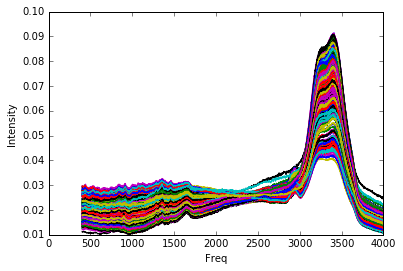Distribution of concentrations¶

In :
plt.hist(concentration, bins=26)
plt.xlabel('Concentration')
print("There are %s different values of concentrations." % np.unique(concentration).size)

There are 21 different values of concentrations.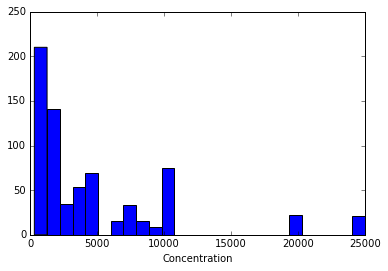In :
for mol in np.unique(molecule):
plt.figure()
plt.hist(concentration[molecule == mol], bins=20)
plt.title(mol + "-  %s values of concentrations."
% np.unique(concentration[molecule == mol]).size)
print(np.unique(concentration[molecule == mol]))

[  300   400   600   800  1000  1400  1600  2000  5000 10000]
[  500  1000  1500  2000  4000  5000  7000 10000 20000 25000]
[ 1000  2000  3000  4000  5000  6000  7000  8000  9000 10000]
[  400   800  1000  1200  1600  2000  3000  4000  5000 10000]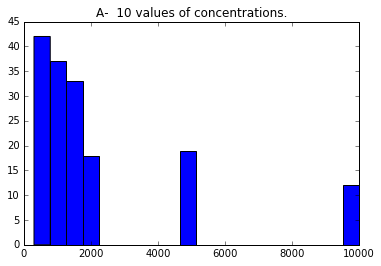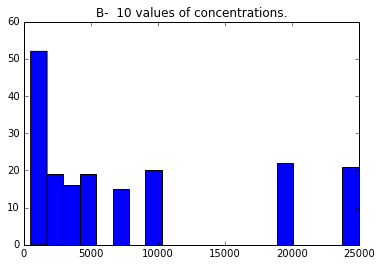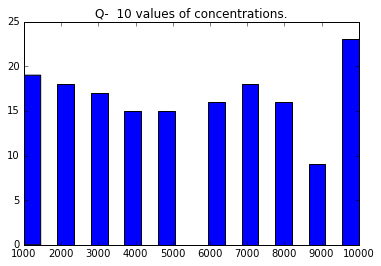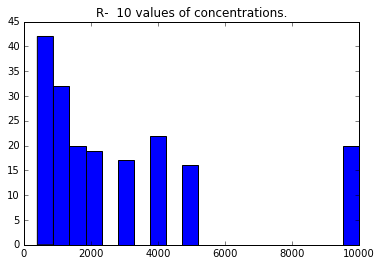Proportion of molecules¶

In :
print('Number of samples: %s' % len(y_df))
y_df.groupby('molecule').count().plot(
y='concentration', kind='pie', autopct='%.2f', figsize=(5, 5));

Number of samples: 699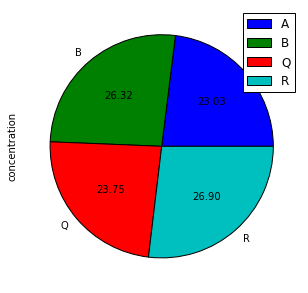Proportion of solute groups and vial group¶

In :
X_df.groupby('solute').count().plot(
y='spectra', kind='pie', autopct='%.2f', figsize=(4, 4), legend=None);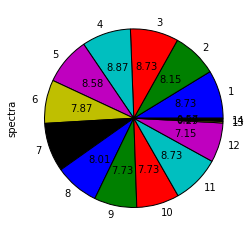In :
X_df.groupby('vial').count().plot(
y='spectra', kind='pie',  autopct='%.2f', figsize=(4, 4), legend=None)

Out:
<matplotlib.axes._subplots.AxesSubplot at 0x1228275d0>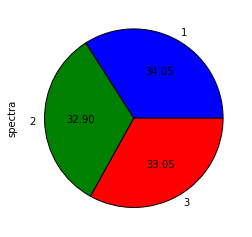Mean Raman spectra for each concentration value¶

In :
for c in np.unique(concentration):
plt.plot(np.mean(spectra[concentration == c, :], axis=0), label="%s" % c)

plt.legend(bbox_to_anchor=(1.2, 1),
bbox_transform=plt.gcf().transFigure);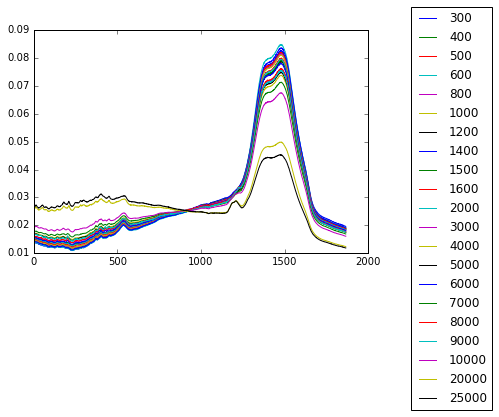Raman spectra for each type of molecule¶

In :
# Mean Raman spectra for each type of molecule
for mol in np.unique(molecule):
plt.plot(np.mean(spectra[molecule == mol, :], axis=0), label="%s" % mol)

plt.legend(bbox_to_anchor=(1.2, 1),
bbox_transform=plt.gcf().transFigure);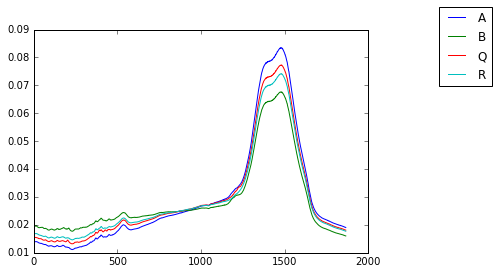In :
# For each molecule
for mol in np.unique(molecule):
plt.figure()
plt.plot(spectra[molecule == mol, :].T)
plt.title(mol + " -  %s samples." % spectra[molecule == mol, :].shape);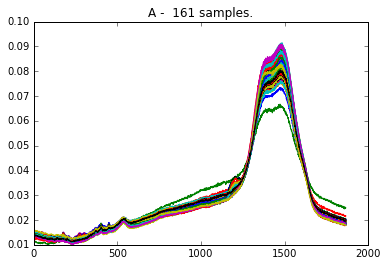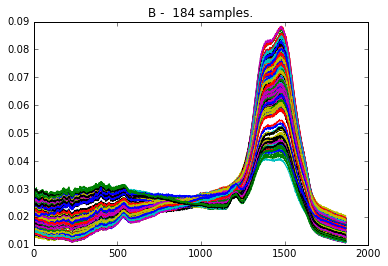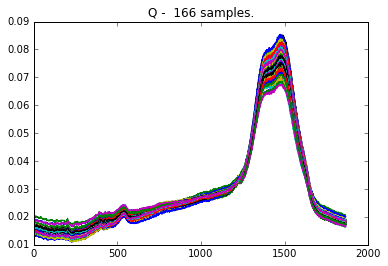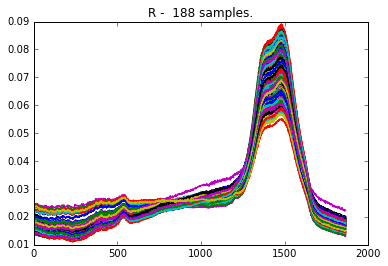The goal of the RAMP is to classify each spectrum into one of the four molecule types, and to measure the concentration of the drugs. The following sketch shows you the four predictive steps you will work on, inserted into the workflow.Each submission will contain four classes, saved in four different files:

• the FeatureExtractor in feature_extractor_clf.py will be used to extract features for classification from the dataset and produce a numpy array.
• the Classifier in classifier.py will predict the molecule type.
• the FeatureExtractor in feature_extractor_reg.py will be used to extract features for regression from the dataset and produce a numpy array.
• the Regressor in regressor.py will predict the drug concentration.

The first step is a feature extractor that takes the raw spectra and the two additional columns in an input data frame, and outputs features in a numpy array that will be used by the molecule classifier. The default feature extractor sends the full raw spectra to the classifier.

Note that the following code cells are not executed in the notebook. The notebook saves their contents in the file specified in the first line of the cell, so you can edit your submission before running the local test below and submitting it at the RAMP site.

In :
%%file submissions/starting_kit/feature_extractor_clf.py
import numpy as np

class FeatureExtractor():
def __init__(self):
pass

def fit(self, X_df, y):
pass

def transform(self, X_df):
X_array = np.array([np.array(dd) for dd in X_df['spectra']])
return X_array

Overwriting submissions/starting_kit/feature_extractor_clf.py


The classifier implements a classifier of the scikit-learn template. The default is a pipeline of a PCA followed by a random forest.

In :
%%file submissions/starting_kit/classifier.py

from sklearn.ensemble import RandomForestClassifier
from sklearn.decomposition import PCA
from sklearn.pipeline import Pipeline
from sklearn.base import BaseEstimator

class Classifier(BaseEstimator):
def __init__(self):
self.n_components = 100
self.n_estimators = 10
self.clf = Pipeline([
('pca', PCA(n_components=self.n_components)),
('clf', RandomForestClassifier(
n_estimators=self.n_estimators, random_state=42))
])

def fit(self, X, y):
self.clf.fit(X, y)

def predict(self, X):
return self.clf.predict(X)

def predict_proba(self, X):
return self.clf.predict_proba(X)

Overwriting submissions/starting_kit/classifier.py


The feature extractor for the concentration regressor will receive the raw spectra and the two additional columns. In addition, since the regressor should know about which molecule it estimates the concentration of, it will also receive four additional columns corresponding to the four molecule types. At train time we send the true label in a one-hot vector, and at test time we send the posterior probabilities that the predict_proba function of the classifier outputs. The default feature extractor normalizes the raw spectra and concatenates the four class posteriors to normalized spectra.

In :
%%file submissions/starting_kit/feature_extractor_reg.py
import numpy as np

label_names = np.array(['A', 'B', 'Q', 'R'])

class FeatureExtractor():
def __init__(self):
pass

def fit(self, X_df, y):
pass

def transform(self, X_df):
X_array = np.array([np.array(dd) for dd in X_df['spectra']])
X_array -= np.median(X_array, axis=1)[:, None]
X_array /= np.sqrt(np.sum(X_array ** 2, axis=1))[:, None]
X_array = np.concatenate([X_array, X_df[label_names].values], axis=1)
return X_array

Overwriting submissions/starting_kit/feature_extractor_reg.py


The default regressor will learn four gradient boosting regressors, one for each molecule type. (Of course, when the classifier makes a mistake, the regressor measures the concentration of the wrong molecule, so the regression performance my also depend on the quality of the classifier.) Another particularity is that we target the logarithm of the concentration (and exponentiate the raw y_pred in predict). This is a common practice when the quality is measured by the relative error.

In :
%%file submissions/starting_kit/regressor.py
from sklearn.decomposition import PCA
from sklearn.pipeline import Pipeline
from sklearn.base import BaseEstimator
import numpy as np

class Regressor(BaseEstimator):
def __init__(self):
self.n_components = 10
self.n_estimators = 40
self.learning_rate = 0.2
self.list_molecule = ['A', 'B', 'Q', 'R']
self.dict_reg = {}
for mol in self.list_molecule:
self.dict_reg[mol] = Pipeline([
('pca', PCA(n_components=self.n_components)),
n_estimators=self.n_estimators,
learning_rate=self.learning_rate,
random_state=42))
])

def fit(self, X, y):
for i, mol in enumerate(self.list_molecule):
ind_mol = np.where(np.argmax(X[:, -4:], axis=1) == i)
X_mol = X[ind_mol]
y_mol = y[ind_mol]
self.dict_reg[mol].fit(X_mol, np.log(y_mol))

def predict(self, X):
y_pred = np.zeros(X.shape)
for i, mol in enumerate(self.list_molecule):
ind_mol = np.where(np.argmax(X[:, -4:], axis=1) == i)
X_mol = X[ind_mol]
y_pred[ind_mol] = np.exp(self.dict_reg[mol].predict(X_mol))
return y_pred

Overwriting submissions/starting_kit/regressor.py


The scores¶

We evaluate the classifier by the classification error and the regression by the mean absolute relative error (MARE)

$$\frac{1}{n}\sum_{k=1}^{n}\left|\frac{y-\hat{y}}{y}\right|.$$

The combined score is a weighted some of these two. Since the classification performance is more important, we weight the classification error by $2/3$ and the MARE by $1/3$.

Local testing (before submission)¶

It is important that you test your submission files before submitting them. For this we provide a unit test. Note that the test runs on your files in submissions/starting_kit.

First pip install ramp-workflow or install it from the github repo. Make sure that the python files feature_extractor_clf.py, classifier.py, feature_extractor_reg.py, and regressor.py are in the submissions/starting_kit folder, and the data train.csv.bz2 and test.csv.bz2 are in data. Then run

ramp_test_submission

If it runs and print training and test errors on each fold, then you can submit the code.

In :
!ramp_test_submission

Testing Drug classification and concentration estimation from Raman spectra
Reading train and test files from ./data ...
Training ./submissions/starting_kit ...
/Users/kegl/anaconda/lib/python2.7/site-packages/pandas/core/indexing.py:337: SettingWithCopyWarning:
A value is trying to be set on a copy of a slice from a DataFrame.
Try using .loc[row_indexer,col_indexer] = value instead

See the caveats in the documentation: http://pandas.pydata.org/pandas-docs/stable/indexing.html#indexing-view-versus-copy
self.obj[key] = _infer_fill_value(value)
/Users/kegl/anaconda/lib/python2.7/site-packages/pandas/core/indexing.py:517: SettingWithCopyWarning:
A value is trying to be set on a copy of a slice from a DataFrame.
Try using .loc[row_indexer,col_indexer] = value instead

See the caveats in the documentation: http://pandas.pydata.org/pandas-docs/stable/indexing.html#indexing-view-versus-copy
self.obj[item] = s
CV fold 0
train combined = 0.012
valid combined = 0.199
test combined = 0.198
train err = 0.002
valid err = 0.157
test err = 0.183
train mare = 0.034
valid mare = 0.284
test mare = 0.226
CV fold 1
train combined = 0.013
valid combined = 0.281
test combined = 0.241
train err = 0.0
valid err = 0.271
test err = 0.233
train mare = 0.039
valid mare = 0.299
test mare = 0.255
CV fold 2
train combined = 0.013
valid combined = 0.275
test combined = 0.217
train err = 0.002
valid err = 0.286
test err = 0.197
train mare = 0.036
valid mare = 0.254
test mare = 0.257
CV fold 3
train combined = 0.012
valid combined = 0.212
test combined = 0.24
train err = 0.0
valid err = 0.236
test err = 0.237
train mare = 0.037
valid mare = 0.165
test mare = 0.246
CV fold 4
train combined = 0.014
valid combined = 0.206
test combined = 0.238
train err = 0.002
valid err = 0.221
test err = 0.25
train mare = 0.037
valid mare = 0.174
test mare = 0.215
CV fold 5
train combined = 0.014
valid combined = 0.307
test combined = 0.264
train err = 0.002
valid err = 0.336
test err = 0.273
train mare = 0.038
valid mare = 0.25
test mare = 0.245
CV fold 6
train combined = 0.013
valid combined = 0.197
test combined = 0.2
train err = 0.002
valid err = 0.2
test err = 0.193
train mare = 0.035
valid mare = 0.191
test mare = 0.213
CV fold 7
train combined = 0.017
valid combined = 0.168
test combined = 0.223
train err = 0.007
valid err = 0.15
test err = 0.207
train mare = 0.038
valid mare = 0.204
test mare = 0.256
----------------------------
train combined = 0.014 ± 0.002
train err = 0.002 ± 0.002
train mare = 0.037 ± 0.002
valid combined = 0.231 ± 0.047
valid err = 0.232 ± 0.06
valid mare = 0.228 ± 0.048
test combined = 0.228 ± 0.021
test err = 0.222 ± 0.029
test mare = 0.239 ± 0.017


Alternatively, load and execute rampwf.utils.testing.py, and call assert_submission. This may be useful if you would like to understand how we instantiate the workflow, the scores, the data connectors, and the cross validation scheme defined in problem.py, and how we insert and train/test your submission. To understand the workflow thoroughly, we suggest that you take a look at drug spectra workflow implementation which shows you the details of training and testing your models.

In :
# %load https://raw.githubusercontent.com/paris-saclay-cds/ramp-workflow/master/rampwf/utils/testing.py

In :
# assert_submission()

Testing Drug classification and concentration estimation from Raman spectra
Reading train and test files from .//data ...
Training .//submissions/starting_kit ...

/Users/kegl/anaconda/lib/python2.7/site-packages/pandas/core/indexing.py:288: SettingWithCopyWarning:
A value is trying to be set on a copy of a slice from a DataFrame.
Try using .loc[row_indexer,col_indexer] = value instead

See the caveats in the documentation: http://pandas.pydata.org/pandas-docs/stable/indexing.html#indexing-view-versus-copy
self.obj[key] = _infer_fill_value(value)
/Users/kegl/anaconda/lib/python2.7/site-packages/pandas/core/indexing.py:465: SettingWithCopyWarning:
A value is trying to be set on a copy of a slice from a DataFrame.
Try using .loc[row_indexer,col_indexer] = value instead

See the caveats in the documentation: http://pandas.pydata.org/pandas-docs/stable/indexing.html#indexing-view-versus-copy
self.obj[item] = s

CV fold 0
train combined = 0.006
valid combined = 0.175
test combined = 0.185
train error = 0.0
valid error = 0.114
test error = 0.147
train mare = 0.017
valid mare = 0.295
test mare = 0.262
CV fold 1
train combined = 0.006
valid combined = 0.182
test combined = 0.178
train error = 0.0
valid error = 0.15
test error = 0.137
train mare = 0.019
valid mare = 0.246
test mare = 0.262
CV fold 2
train combined = 0.006
valid combined = 0.197
test combined = 0.19
train error = 0.0
valid error = 0.157
test error = 0.157
train mare = 0.019
valid mare = 0.277
test mare = 0.258
CV fold 3
train combined = 0.006
valid combined = 0.166
test combined = 0.159
train error = 0.0
valid error = 0.136
test error = 0.123
train mare = 0.019
valid mare = 0.226
test mare = 0.229
CV fold 4
train combined = 0.006
valid combined = 0.13
test combined = 0.159
train error = 0.0
valid error = 0.1
test error = 0.117
train mare = 0.019
valid mare = 0.189
test mare = 0.245
CV fold 5
train combined = 0.007
valid combined = 0.177
test combined = 0.173
train error = 0.0
valid error = 0.15
test error = 0.14
train mare = 0.02
valid mare = 0.232
test mare = 0.239
CV fold 6
train combined = 0.006
valid combined = 0.127
test combined = 0.185
train error = 0.0
valid error = 0.086
test error = 0.15
train mare = 0.019
valid mare = 0.208
test mare = 0.254
CV fold 7
train combined = 0.007
valid combined = 0.154
test combined = 0.19
train error = 0.0
valid error = 0.114
test error = 0.15
train mare = 0.02
valid mare = 0.232
test mare = 0.271
----------------------------
train combined = 0.006 ± 0.0003
train error = 0.0 ± 0.0
train mare = 0.019 ± 0.0008
valid combined = 0.163 ± 0.0234
valid error = 0.126 ± 0.0245
valid mare = 0.238 ± 0.0323
test combined = 0.178 ± 0.0119
test error = 0.14 ± 0.013
test mare = 0.253 ± 0.0127
----------------------------
Testing if the notebook can be converted to html


Submitting to ramp.studio¶

Once you found a good model, you can submit it to ramp.studio. First, if it is your first time using RAMP, sign up, otherwise log in. Then find an open event on the particular problem, for example, the event drug spectra for this RAMP. Sign up for the event. Both signups are controled by RAMP administrators, so there can be a delay between asking for signup and being able to submit.

Once your signup request is accepted, you can go to your sandbox and copy-paste (or upload) feature_extractor_clf.py, and classifier.py, feature_extractor_reg.py, and regressor.py from submissions/starting_kit. Save it, rename it, then submit it. The submission is trained and tested on our backend in the same way as ramp_test_submission does it locally. While your submission is waiting in the queue and being trained, you can find it in the "New submissions (pending training)" table in my submissions. Once it is trained, you get a mail, and your submission shows up on the public leaderboard. If there is an error (despite having tested your submission locally with ramp_test_submission), it will show up in the "Failed submissions" table in my submissions. You can click on the error to see part of the trace.

After submission, do not forget to give credits to the previous submissions you reused or integrated into your submission.

The data set we use at the backend is usually different from what you find in the starting kit, so the score may be different.

The usual way to work with RAMP is to explore solutions, add feature transformations, select models, perhaps do some AutoML/hyperopt, etc., locally, and checking them with ramp_test_submission. The script prints mean cross-validation scores

----------------------------
train combined = 0.006 ± 0.0004
train error = 0.0 ± 0.0
train mare = 0.019 ± 0.0011
valid combined = 0.17 ± 0.024
valid error = 0.135 ± 0.0298
valid mare = 0.24 ± 0.0259
test combined = 0.177 ± 0.015
test error = 0.142 ± 0.0203
test mare = 0.248 ± 0.0137

The official score in this RAMP (the first score column after "historical contributivity" on the leaderboard) is a weighted combination of "error" and "mare" ("combined"), so the line that is relevant in the output of ramp_test_submission is valid mare = 0.24 ± 0.0259. When the score is good enough, you can submit it at the RAMP.